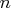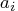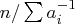Previous Page | Next Page

 Language Reference

## HARMEAN Function

calculates harmonic means

HARMEAN( matrix)

where matrix is a numeric matrix of nonnegative values.

The HARMEAN function returns a scalar containing the harmonic mean of the elements of the input matrix. The harmonic mean of a set of positive numbersisdivided by the sum of the reciprocals of. That is,.

The harmonic mean is zero if any of theare zero. The harmonic mean is not defined for negative inputs. If any of theare missing, they are excluded from the computation.

The harmonic mean is sometimes used to compute an average sample size in an unbalanced experimental design. For example, the following statements compute an average sample size for five samples:


sizes = { 8, 12, 23, 10, 8 }; /* sample sizes */
aveSize = harmean( sizes );
print aveSize;

aveSize

10.486322Previous Page | Next Page | Top of Page